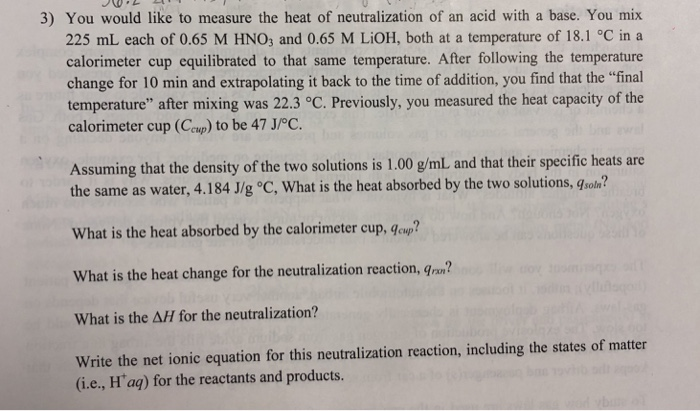# 3) You would like to measure the heat of neutralization of an acid with a base....

###### Question:3) You would like to measure the heat of neutralization of an acid with a base. You mix 225 mL each of 0.65 M HNO, and 0.65 M LiOH, both at a temperature of 18.1 °C in a calorimeter cup equilibrated to that same temperature. After following the temperature change for 10 min and extrapolating it back to the time of addition, you find that the "final temperature" after mixing was 22.3 °C. Previously, you measured the heat capacity of the calorimeter cup (Ceup) to be 47 JPC Assuming that the density of the two solutions is 1.00 g/ml and that their specific heats are the same as water, 4.184 J/g C, What is the heat absorbed by the two solutions, sola? What is the heat absorbed by the calorimeter cup, gcup? What is the heat change for the neutralization reaction, gra? What is the AH for the neutralization? Write the net ionic equation for this neutralization reaction, including the states of matter (i.e., H'aą) for the reactants and products.

#### Similar Solved Questions

##### 3.) Three particles of charge -4.50 nC, +4.50 nC, and +7.20 nC, respectively, are each traveling...
3.) Three particles of charge -4.50 nC, +4.50 nC, and +7.20 nC, respectively, are each traveling at a speed of 2.25x10 m/s when they enter a uniform magnetic field as shown in the picture. If the magnetic field strength is 0.500 T, find the magnitude and direction of the force exerted on each charge...
##### Classify each resource used in production as land, labor, capital, or entrepeneurs. Land Labor Capital Entrepreneurs...
Classify each resource used in production as land, labor, capital, or entrepeneurs. Land Labor Capital Entrepreneurs Answer Bank Aunt Sandy's Jammin' Jellies (the business) Anika, a server at Sonic Drive-In your favorite bartender We were unable to transcribe this image...
##### Given that A, B, and C are true and X, Y, and Z are false, determine...
Given that A, B, and C are true and X, Y, and Z are false, determine the truth value of the following statement: [(A v B) & ~(Y & ~C)] v ~[~(Z --> ~X) v ~B] True False...
##### AgC,H,O, (aq) + BaCl(aq) → Express your answer as a chemical equation. Identify all of the...
AgC,H,O, (aq) + BaCl(aq) → Express your answer as a chemical equation. Identify all of the phases in your answer. Enter noreaction if there is no reaction. - AED ROO? Submit Previous Answers Request Answer X Incorrect; Try Again; 5 attempts remaining Part B BaCl(aq) + K, CO3(aq) → Express ...
##### Write a C program that includes a function called moveEm() that accepts the ADDRESSES of three...
Write a C program that includes a function called moveEm() that accepts the ADDRESSES of three double variables as input. The function should move the value of the smallest variable into the first variable, the middle value into the middle variable, and the largest value into the third variable. Cal...
##### Refer to the classification of drugs and poisons and interpret the following schedules of drugs (in...
Refer to the classification of drugs and poisons and interpret the following schedules of drugs (in 10-20 words each) and provide two (2) examples for each schedule. Schedule Interpretation(category of drug/poison) Two examples 8.1) 2 8.2) 3 8.3) 4 8.4) 8...
##### At 30 degrees North, is the evaporation rate positive or negative? Meaning is there more evaporat...
At 30 degrees North, is the evaporation rate positive or negative? Meaning is there more evaporation than precipitation, and if so why?...
##### This is a java program that runs on the Eclipse. Java Programming 2-1: Java Class Design...
This is a java program that runs on the Eclipse. Java Programming 2-1: Java Class Design Interfaces Practice Activities Lesson objectives: Model business problems using Java classes Make classes immutable User Interfaces Vocabulary: Identify the vocabulary word for each definition below. A speciali...